Formulae_Index
Nomenclature

Stress/Strain Formulae

Basic Definitions

Note: For more detailed stress & strain notes refer to webpage Stress & Strain

Strain = Change in length (dL)over original length (L)

e = dL / L

Stress = Force (F) divided by Area withstanding Force (A)

σ = F / A

Young's Modulus E = Stress ( s ) / Strain(e). This is a property of a material

E = s / e

Bending

General Formula for Bending

A beam with a moment of inertia I and with Young's modulus E will have a bending stress f at a distance from the Neutral Axis (NA) y and the NA will bend to a radius R ...in accordance with the following formula.

M / I = s / y = E / R

Important note
W and w as used below for beam concentrated load, total load and uniform distributed load are assumed to be in units of force i.e. Newtons   If they are provided in units of weight i.e kg then they should be converted into units of force by mutliplying by the gravity constant g (9.81)

Simply Supported Beam . Concentrated Load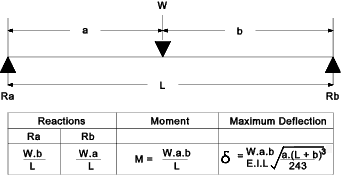Simply Supported Beam . Uniformly Distributed Load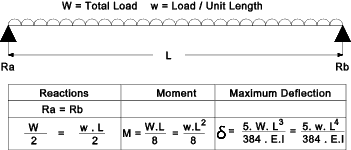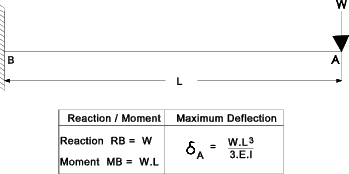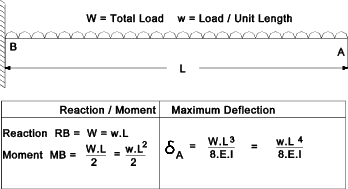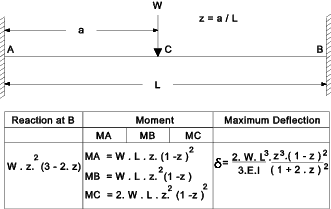Fixed Beam . Uniformly Distributed Load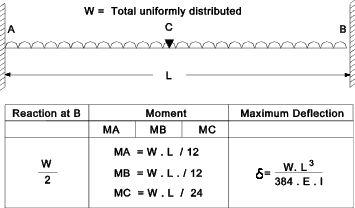Torsion /Shear

Poisson's Ratio = ν = (lateral strain / primary strain )

Shear Modulus G = Shear Stress /Shear Strain

G = τ / ε = E / (2 .( 1 + ν ))

General Formula for Torsion

A shaft subject to a torque T having a polar moment of inertia J and a shear Modulus G will have a shear stress q at a radius r and an angular deflection θ over a length L as calculated from the following formula.

T / J = G . θ / L = t / r

More detailed notes on torsion calculations are found at webpage Torsion

Pressure Vessels - Thin Walled Cylinders

For a thin walled cylinder subject to internal pressure P the circumferential stress = σc

This stress tends to stretch the cylinder along its length. This is also called the longitudinal stress.

σc = P . d / ( 4 . t )

For a thin walled cylinder subject to internal pressure P the tangential stress = σc
This stress tends to increase the diameter). This is also called the hoop stress.

σt = P . d / ( 2 . t )

The above two formulae are only valid if the ratio of thickness to dia is less than 1:20

Pressure Vessels - Thick Walled Cylinders

The equations for the stresses in thick walled cylinders are derived on web page Cylinders

p1 = internal pressure
p2 = external pressure
σ t =tangential stress

Consider a cylinder with and internal diameter d 1, subject to an internal pressure p 1.  The external diameter is d 2 which is subject to an external pressure p 2.    The radial pressures at the surfaces are the same as the applied pressures therefore

σ r = A + B / r 2
σ t = A - B / r 2

The radial pressures at the surfaces are the same as the applied pressures therefore

- p1 = A + B / r 12
-p2 = A + B / r 22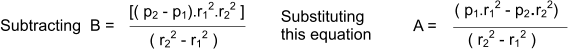The resulting general equations are known as Lame's Euqations and are shown as follows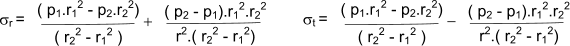If the external pressure is zero this reduces to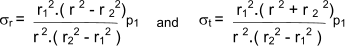If the internal pressure is zero this reduces to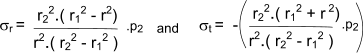Formulae_Index
Nomenclature